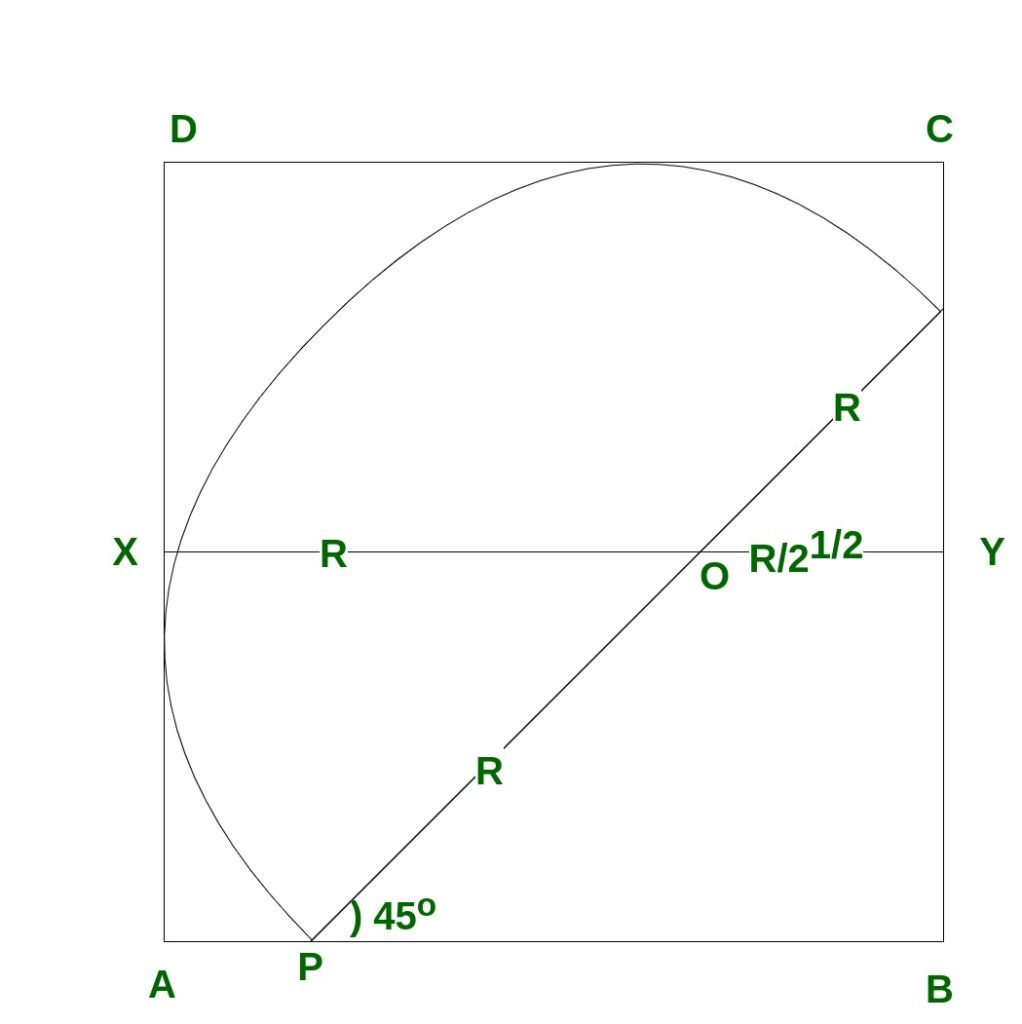Open in App
Not now

# Area of largest semicircle that can be drawn inside a square

• Last Updated : 21 Jun, 2022

Given a square of side a, the task is to find the area of the largest semi-circle that can be drawn inside the square.

Examples:

```Input: a = 3
Output: 4.84865

Input: a = 4
Output: 8.61982```

Approach
The semicircle of maximal area inscribed in the square has its diameter parallel to a diagonal, and its radius rmax is given as:• Since the figure is symmetrical in the diagonal BD, angle QPB = 45°
`OY = r cos 45 = r/ √2`
• Hence
```a = AB
= r + r/√2
= r(1 + 1/√2)```
• Thus
```r = a / (1 + 1/√2)
= a*√2 / (√2 + 1)```
• Rationalizing the denominator, we obtain
`r = a*√2*(√2-1)`
• Thus
```r = a*2 - a √2
= a*(2-√2)```
• Therefore,
```Area of the required semicircle
= pi * r2/2
= 3.14*(a*(2-√2))2 / 2```
• Below is the implementation of the above approach:

## CPP

 `// C++ program to find Area of``// semicircle in a square` `#include ``using` `namespace` `std;` `// Function to find area of semicircle``float` `find_Area(``float` `a)``{``    ``float` `R = a * (2.0 - ``sqrt``(2));``    ``float` `area = 3.14 * R * R / 2.0;``    ``return` `area;``}` `// Driver code``int` `main()``{``    ``// side of a square``    ``float` `a = 4;` `    ``// Call Function to find``    ``// the area of semicircle``    ``cout << ``" Area of semicircle = "``         ``<< find_Area(a);` `    ``return` `0;``}`

## Java

 `// Java program to find Area of``// semicircle in a square``class` `GFG {` `    ``// Function to find area of semicircle``    ``static` `float` `find_Area(``float` `a)``    ``{``        ``float` `R = a * (``float``)(``2.0` `- Math.sqrt(``2``));``        ``float` `area = (``float``)((``3.14` `* R * R) / ``2.0``);``        ``return` `area;``    ``}``    ` `    ``// Driver code``    ``public` `static` `void` `main (String[] args)``    ``{``        ``// side of a square``        ``float` `a = ``4``;``    ` `        ``// Call Function to find``        ``// the area of semicircle``        ``System.out.println(``" Area of semicircle = "` `+ find_Area(a));``    ``}``}` `// This code is contributed by AnkitRai01`

## Python3

 `# Python3 program to find Area of``# semicircle in a square``from` `math ``import` `sqrt` `# Function to find area of semicircle``def` `find_Area(a) :` `    ``R ``=` `a ``*` `(``2.0` `-` `sqrt(``2``));``    ``area ``=` `3.14` `*` `R ``*` `R ``/` `2.0``;``    ` `    ``return` `area;` `# Driver code``if` `__name__ ``=``=` `"__main__"` `:` `    ``# side of a square``    ``a ``=` `4``;` `    ``# Call Function to find``    ``# the area of semicircle``    ``print``(``"Area of semicircle ="``,find_Area(a));` `# This code is contributed by AnkitRai01`

## C#

 `// C# program to find Area of``// semicircle in a square``using` `System;` `class` `GFG {` `    ``// Function to find area of semicircle``    ``static` `float` `find_Area(``float` `a)``    ``{``        ``float` `R = a * (``float``)(2.0 - Math.Sqrt(2));``        ``float` `area = (``float``)((3.14 * R * R) / 2.0);``        ``return` `area;``    ``}``    ` `    ``// Driver code``    ``public` `static` `void` `Main (``string``[] args)``    ``{``        ``// side of a square``        ``float` `a = 4;``    ` `        ``// Call Function to find``        ``// the area of semicircle``        ``Console.WriteLine(``" Area of semicircle = "` `+ find_Area(a));``    ``}``}` `// This code is contributed by AnkitRai01`

## Javascript

 ``

Output:

`Area of semicircle = 8.61982`

Time Complexity: O(1)

Auxiliary Space: O(1)

My Personal Notes arrow_drop_up